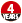# leetcode 169. 多数元素

2020-03-15 18:22 58 查看

# leetcode 169. 多数元素

## 题目详情

*你可以假设数组是非空的，并且给定的数组总是存在多数元素。

• 示例 1:
输入: [3,2,3]
输出: 3
• 示例 2:
输入: [2,2,1,1,1,2,2]
输出: 2

## 我的代码

```class Solution {
public:
int majorityElement(vector<int>& nums) {
int len = nums.size();
if ((len == 1) || (len == 2)) {
return nums;
}
map<int, int> index;
for (int num: nums) {
if (index.count(num) == 0) {
index[num] = 1;
continue;
}
index[num] += 1;
}
for (auto iter = index.begin(); iter != index.end(); ++iter) {
if (iter->second > len / 2) {
return iter->first;
}
}
return nums;
}
};```

### 一些想法

1. 本题最简单的思路莫过于先排序，然后返回处于中间的数字，但如此做下来效果并不是很理想。
2. 改进想法是先遍历数组，然后读入map记录每个元素出现的次数，再遍历map。

## 执行用时为 4 ms 的范例

```class Solution {
public:
int majorityElement(vector<int>& nums) {
int num=nums,count=1;
for(int i=1;i<nums.size();i++){
if(nums[i]==num) count++;
else{
count--;
if(count<0){
num=nums[i];
count=1;
}
}}
return num;
}
};```

### 思考

• 点赞
• 收藏
• 分享
• 文章举报摸鱼混子 发布了6 篇原创文章 · 获赞 0 · 访问量 40 私信 关注